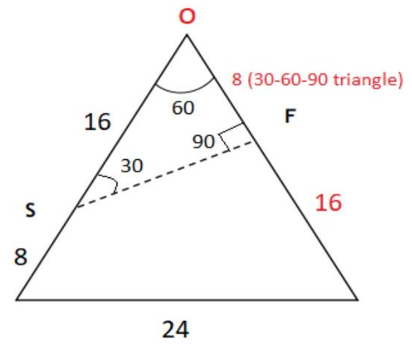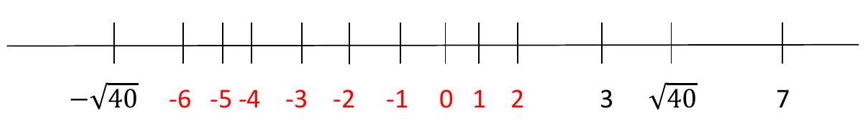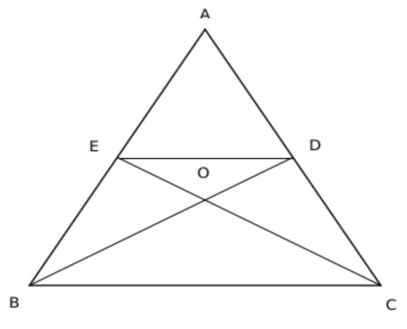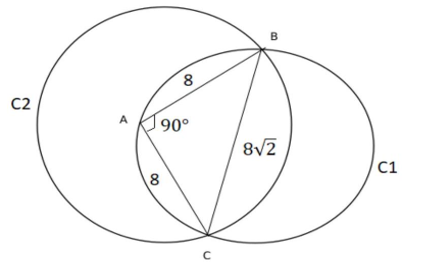Bodhee Prep-Online CAT Coaching | Online CAT Preparation | CAT Online Courses

Get 10% OFF on CAT 24 Course. Code: BODHEE10. valid till 3rd Dec Enroll Now
| Best Online CAT PreparationFor Enquiry CALL @ +91-95189-40261

# CAT 2022 Quant Questions with Solutions (Slot 3)

Question 1:

The arithmetic mean of all the distinct numbers that can be obtained by rearranging the digits in 1421, including itself, is

1. 3333

2. 2442

3. 2222

4. 2592

Option: 3
Explanation:

The number of 4 digit numbers possible using $1,1,2$, and 4 is $\frac{4 !}{2 !}=12$

There will be 6 (3!) numbers with 1 at the unit's place, $3\left(\frac{3 !}{2 !}\right)$ numbers each with 2 or 4 at the unit's place

Number of $1^{\prime}$ s, 2's and $4^{\prime}$ s in units digits will be in the ratio 2: 1:1, i.e. $61^{\prime}$ s, $32^{\prime}$ s and $34^{\prime}$ s.

Sum $=6(1)+3(2)+3(4)=24$

Similarly, in tens digit, hundreds digit and thousands digit as well.

Therefore, sum $=24(1)+24(10)+24(100)+24(1000)=24(1111)$

Mean $=\frac{24(1111)}{12}=2222$

Question 2:

Two ships are approaching a port along straight routes at constant speeds. Initially, the two ships and the port formed an equilateral triangle with sides of length 24 km. When the slower ship travelled 8 km, the triangle formed by the new positions of the two ships and the port became right-angled. When the faster ship reaches the port, the distance, in km, between the other ship and the port will be

1. 6

2. 4

3. 8

4. 12

Option: 4
Explanation:Let $S$ be the slower ship and $F$ be the faster ship.

It is given that when $S$ travelled $8 \mathrm{~km}$, the positions of ships with the port is forming a right triangle.

Since one of the angles is $60^{\circ}$ (since one vertex is still part of the equilateral triangle),

the other two vertices will have angles of 30 and 90.

The distance between $O$ and $S=24-8=16$

Thus, $O F=8$. Thus in the time, $S$ covered $8 \mathrm{~km}, F$ will cover $24-8=16 \mathrm{~km}$.

Thus, the ratio of their speeds faster ship to slower ship is $2: 1$,

Thus, when $F$ covers $24 \mathrm{~km}, S$ will cover $12 \mathrm{~km}$.

Question 3:

Suppose k it is any integer such that the equation 2x2 + kx + 5 = 0 has no real roots and the equation x2 + (k - 5)x + 1 = 0 has two distinct real roots for x. Then, the number of possible values of k is

1. 9

2. 13

3. 8

4. 7

Option: 1
Explanation:

$2 x^{2}+k x+5=0 \text { has no real roots, }$

$k^{2}-40<0,$

$k^{2}<40,$

$-\sqrt{40}<k<\sqrt{40},-----(1)$

$x^{2}+(k-5) x+1=0 \text { has } 2 \text { distinct real roots, }$

$(k-5)^{2}-4>0,$

$(k-5)^{2}>4,$

$k-5<-2 \text { or } k-5>2,$

$k<3$ or $k>7,----(2)$

Combining (1) and (2), $\quad-\sqrt{40}<k<3$9 integer values $\{-6,-5,-4,-3,-2,-1,0,1,2\}$

Question 4:

A glass contains 500 cc of milk and a cup contains 500 cc of water. From the glass, 150 cc of milk is transferred to the cup and mixed thoroughly. Next, 150 cc of this mixture is transferred from the cup to the glass. Now, the amount of water in the glass and the amount of milk in the cup are in the ratio

1. 3 : 10

2. 10 : 3

3. 1 : 1

4. 10 : 13

Option: 3
Explanation:

Initially: a glass 500cc milk and a cup 500cc water

Step 1: 150 cc of milk is transferred to the cup from glass

After step 1: Glass - 350 cc milk, Cup - 150 cc milk and 500 cc water

Step 2: 150 cc of this mixture is transferred from the cup to the glass After step 2:

Glass $-350 c c$ milk $+150$ cc mixture with milk : water ratio 3: 10

Cup - 500 cc mixture with milk : water ratio $3: 10$

water in glass : milk in cup $=\frac{10}{13} \times 150: \frac{3}{13} \times 500=1: 1$

Question 5:

If $c=\frac{16 x}{y}+\frac{49 y}{x}$ for some non-zero real numbers x and y, then c cannot take the value

1. 60
2. -50
3. -70
4. -60

Option: 2
Explanation:

Let $\cfrac{x}{y}=k$

Therefore, $c=16 k+\cfrac{49}{k}$

Applying $A M \geq G M$

$\cfrac{16 k+\cfrac{49}{k}}{2} \geq \sqrt{16 k \times \cfrac{49}{k}}$ $16 k+\cfrac{49}{k} \geq 56$

When $\mathrm{k}$ is positive then $\mathrm{c}$ is greater than equal to 56 .

When $\mathrm{k}$ is negative then $\mathrm{c}$ is less than equal to $-56$.

Therefore, $c \leq-56$ or $c \geq 56$

$c$ can't be $-50$

Question 6:

The minimum possible value of $\frac{x^2-6 x+10}{3-x}$ , for x < 3, is

1. -1/2

2. 1/2

3. 2

Option: 4
Explanation:

$\cfrac{x^{2}-6 x+10}{3-x}=(3-x)+\cfrac{1}{(3-x)}($ by long division method $)$

as $x<3$

$3-x>0$

$\left(A+\cfrac{1}{A}\right) \geq 2$, if $A>0$ $(3-x)+\cfrac{1}{(3-x)} \geq 2$

Question 7:

Bob can finish a job in 40 days, if he works alone. Alex is twice as fast as Bob and thrice as fast as Cole in the same job. Suppose Alex and Bob work together on the first day, Bob and Cole work together on the second day, Cole and Alex work together on the third day, and then, they continue the work by repeating this three-day roster, with Alex and Bob working together on the fourth day, and so on. Then, the total number of days Alex would have worked when the job gets finished, is

Option: 11
Explanation:

Let the efficiency of Bob be 3 units/day. So, Alex's efficiency will be 6 units/day, and Cole's will be 2 units/day.

Since Bob can finish the job in 40 days, the total work will be $40 \times 3=120$ units.

Since Alex and Bob work on the first day, the total work done $=3+6=9$ units.

Similarly, for days 2 and 3, it will be 5 and 8 units, respectively.

Thus, in the first 3 days, the total work done $=9+5+8=22$ units.

The work done in the first 15 days $=22 \times 5=110$ units.

16 th day work $=9$ units

Total work on 16 th day $=119$ units Then he will also work on the 16 th day.

The total number of days Alex would have worked $=11$.

Question 8:

Moody takes 30 seconds to finish riding an escalator if he walks on it at his normal speed in the same direction. He takes 20 seconds to finish riding the escalator if he walks at twice his normal speed in the same direction. If Moody decides to stand still on the escalator, then the time, in seconds, needed to finish riding the escalator is

Option: 60
Explanation:

Let the speed of Moody be ' $x^{\prime}$ steps/sec and that of the escalator be ' $e^{\prime}$ steps/sec.

In 30 seconds, Moody will finish riding the escalator when going in the same direction.

Thus, total steps $=30(x+e)$

If Moody's speed becomes twice, the time becomes 20 seconds.

Thus, total steps $=20(2 x+e)$

Or $30 x+30 e=40 x+20 e$

Or

$x=y$

So, total steps $=60 e$

Time taken by only escalator $=\cfrac{60 e}{e}=60$ seconds

Question 9:

Consider six distinct natural numbers such that the average of the two smallest numbers is 14, and the average of the two largest numbers is 28. Then, the maximum possible value of the average of these six numbers is

1. 23

2. 24

3. 23.5

4. 22.5

Option: 4
Explanation:

Let the six numbers be $a, b, c, d, e, f$ in ascending order

$a+b=28$

$e+f=56$ If we want to maximise the average then we have to minimise $a, b$, maximise $c, d$, $e$ and minimise $f$

(do remember the numbers are in ascending order)

$a+b=28=13+15$

$e+f=56=27+29$

As $a, b$ e and $f$ are distinct natural numbers

Therefore $c$ and $d$ will be 25 and 26 respecitively

So average $=\cfrac{a+b+c+d+e+f}{6}=\cfrac{28+25+26+56}{6}=22.5$

Question 10:

The lengths of all four sides of a quadrilateral are integer valued. If three of its sides are of length 1 cm, 2 cm and 4 cm, then the total number of possible lengths of the fourth side is

1. 5

2. 4

3. 3

4. 6

Option: 1
Explanation:

Sum of the three sides of a quadrilateral is greater than the fourth side. Therefore, let the fourth side be $x$

$1+2+4>x$ or $x<7$

$1+2+x>4$ or $x>1$

Possible values of $x$ are $2,3,4,5$ and 6 .

Question 11:

The average of all 3-digit terms in the arithmetic progression 38, 55, 72, ..., is

Option: 548
Explanation:

General term $=38+(n-1) 17=17 n+21=17(n+1)+4=17 k+4$

Each term is in the form of $17 k+4$

Least 3 - digit number in the form of $17 k+4$ is at $k=6$, i.e. 106

Highest 3 - digit number in the form of $17 k+4$ is at $k=58$, i.e. 990 Average of an A.P. $=\cfrac{\text { first term }+\text { last term }}{2}=\cfrac{106+990}{2}=548$

Question 12:

If $\left(\sqrt{\frac{7}{5}}\right)^{3 x-y}=\frac{875}{2401}$ and $\left(\frac{4 a}{b}\right)^{6 x-y}=\left(\frac{2 a}{b}\right)^{y-6 x}$ , for all non-zero real values of a and b, then the value of x + y is

Option: 14
Explanation:

$\left(\cfrac{\sqrt{7}}{5}\right)^{3 x-y}=\cfrac{875}{2401}$ and $\left(\cfrac{4 a}{b}\right)^{6 x-y}=\left(\cfrac{2 a}{b}\right)^{y-6 x}$

$\left(\cfrac{7}{5}\right)^{\cfrac{3 x-y}{2}}=\cfrac{125}{343}$

$\left(\cfrac{7}{5}\right)^{\cfrac{3 x-y}{2}}=\left(\cfrac{7}{5}\right)^{-3}$

$3 x-y=-6$

$\left(\cfrac{4 a}{b}\right)^{6 x-y}=\left(\cfrac{2 a}{b}\right)^{y-6 x}$

Therefore,

$y=6 x$

as the bases are different so the power should be zero for the results to be equal.

$3 x-y=-6$

$\text { or, } 3 x-6 x=-6$

$\text { or } x=2$

$y=6 x=12$

$x+y=14$

Question 13:

Suppose the medians BD and CE of a triangle ABC intersect at a point O. If area of triangle ABC is 108 sq. cm., then, the area of the triangle EOD, in sq. cm., is

Option: 9
Explanation:Area of $A B D:$ Area of $B D C=1: 1$

Therefore, area of $A B D=54$

Area of ADE : Area of $E D B=1: 1$

Therefore, area of $A D E=27=E D B$ $O$ is the centroid and it divides the medians in the ratio of $2: 1$

Area of $B E O:$ Area of $E O D=2: 1$ (Triangles with same height will have areas in the ratio of their bases)

Area of $E O D=\left(\cfrac{1}{3} \times 27\right)=9$

Question 14:

A donation box can receive only cheques of ₹100, ₹250, and ₹500. On one good day, the donation box was found to contain exactly 100 cheques amounting to a total sum of ₹15250. Then, the maximum possible number of cheques of ₹500 that the donation box may have contained, is

Option: 12
Explanation:

Let the number of 100 cheques, 250 cheques and 500 cheques be $x, y$ and $z$ respectively, $\quad x, y, z$ be whole numbers

We need to find the maximum value of $z$.

$x+y+z=100 \ldots \ldots(1)$

$100 x+250 y+500 z=15250$

$2 x+5 y+10 z=305 \ldots \ldots$ (2)

multiply (1) by 2 $2 x+2 y+2 z=200 \ldots(3)$

$(5)-(3)$

$3 y+8 z=105, z=\cfrac{105-3 y}{8}$

To maximise z, minimise y such that $105-3 y$ is a multiple of 8

$y=3, z=12$ is the maximum value

Therefore, maximum number of 500 notes is 12.

Question 15:

Two cars travel from different locations at constant speeds. To meet each other after starting at the same time, they take 1.5 hours if they travel towards each other, but 10.5 hours if they travel in the same direction. If the speed of the slower car is 60 km/hr, then the distance traveled, in km, by the slower car when it meets the other car while traveling towards each other, is

1. 100

2. 90

3. 120

4. 150

Option: 2
Explanation:

Both the cars take $1.5$ hrs to meet when they travel towards each other.

It is given, speed of slower car is $60 \mathrm{~km} / \mathrm{hr}$

Therefore, distance covered by slower car when they meet $=60 \times 1.5=90 \mathrm{~km}$

Question 16:

A school has less than 5000 students and if the students are divided equally into teams of either 9 or 10 or 12 or 25 each, exactly 4 are always left out. However, if they are divided into teams of 11 each, no one is left out. The maximum number of teams of 12 each that can be formed out of the students in the school is

Option: 150
Explanation:

Since the total number of students, when divided by either 9 or 10 or 12 or 25 each, gives a remainder of 4 , the number will be in the form of $\operatorname{LCM}(9,10,12,25) k+4=900 k+4$.

It is given that the value of $900 k+4$ is less than 5000.Also, it is given that $900 k+4$ is divided by 11 .

It is only possible when $k=2$ and total students $=1804$.

So, the number of 12 students group $=\cfrac{1800}{12}=150$

Question 17:

Nitu has an initial capital of ₹20,000. Out of this, she invests ₹8,000 at 5.5% in bank A, ₹5,000 at 5.6% in bank B and the remaining amount at x% in bank C, each rate being simple interest per annum. Her combined annual interest income from these investments is equal to 5% of the initial capital. If she had invested her entire initial capital in bank C alone, then her annual interest income, in rupees, would have been

1. 800

2. 700

3. 900

4. 1000

Option: 1
Explanation:

$5.5 \%$ of $8000+5.6 \%$ of $5000+x \%$ of $7000=5 \%$ of 20000 ,

$x=4$,

$4 \%$ of $20000=800$,

Question 18:

In a triangle ABC, AB = AC = 8 cm. A circle drawn with BC as diameter passes through A. Another circle drawn with center at A passes through B and C. Then the area, in sq. cm, of the overlapping region between the two circles is

1. $16(\pi-1)$
2. $32(\pi$
3. $16\pi$
4. $32(\pi-1)$

Option: 4
Explanation:Area of circle $C 1=\pi(4 \sqrt{2})^{2}=32 \pi$

option 4 is the correct answer $32(\pi-1)$

If the options are not there, then

overlapping area $=\cfrac{1}{2}($ Area of circle C1 $)+$ Area of the minor sector made by $B C$ in $C 2=16 \pi+\cfrac{1}{4} 64 \pi-\cfrac{1}{2}(8)^{2} \sin 90^{\circ}=32(\pi-1)$

Question 19:
Let $r$ be a real number and $f(x)=\left\{\begin{array}{cl}2 x-r & \text { if } x \geq r \\ r & \text { if } x < r\end{array}\right.$. Then, the equation $f(x)=f(f(x))$ holds for all real values of $x$ where
1. $x \neq r$
2. $x>r$
3. $x \geq r$
4. $x \leq r$

Option: 4
Explanation:

When $x<r$,

$f(x)=r$

$f(x)=f(f(x))$

$r=f(r)$,

$r=2 r-r$,

$r=r$

When $x=r$,

$f(x)=2 x-r=2 r-r=r$,

$f(x)=f(f(x))$,

$r=f(r)$,

$r=2 r-r$, $r=r$

Therefore $x \leq r$

Question 20:

A group of N people worked on a project. They finished 35% of the project by working 7 hours a day for 10 days. Thereafter, 10 people left the group and the remaining people finished the rest of the project in 14 days by working 10 hours a day. Then the value of N is

1. 150

2. 36

3. 23

4. 140

Option: 4
Explanation:

Let the unit of work done by 1 man in 1 hour and 1 day be 1 MDH unit (Man Day Hour).

Thus, in 7 hours per day for 10 days, the work done by $N$ people $=N \times 7 \times 10$ MDH units. $=7 O N$

Since this is equal to $35 \%$ of the total work,

$35 \%$ of the total work $=70 \mathrm{~N}$

Total work $=\cfrac{(70 N \times 100)}{35}=200 N$

The work left $=200 \mathrm{~N}-70 \mathrm{~N}=130 \mathrm{~N} \mathrm{MDH}$ units.

Now, 10 people left the job. So, the number of people left $=(N-10)$ Since $(N-10)$ people completed the rest of work in 14 days by working 10 hours a day,

$(N-10) \times 14 \times 10=130 N$

$10 N=1400$

$N=140$

Question 21:

If $(3+2 \sqrt{2})$ is a root of the equation $a x^2+b x+c=0$, and $(4+2 \sqrt{3})$ is a root of the equation $a y^2+m y+n=0$, where $a$, $b, c, m$ and $n$ are integers, then the value of $\left(\frac{\boldsymbol{b}}{\boldsymbol{m}}+\frac{\boldsymbol{c}-2 \boldsymbol{b}}{\boldsymbol{n}}\right)$ is

1. 4

2. 0

3. 1

4. 3

Option: 1
Explanation:

$a, b, c, m$ and $n$ are integers so if one root is $3+2 \sqrt{2}$ then the other root is $3-2 \sqrt{2}$

Sum of roots $=6=-b / a$ or $b=-6 a$

Product of roots $=1=c / a$ or $c=a$

$a, b, c, m$ and $n$ are integers so if one root is $4+2 \sqrt{3}$ then the other root is $4-2 \sqrt{3}$ Sum of roots $=8=-m / a$ or $m=-8 a$

product of roots $=4=n / a$ or $n=4 a$

$\left(\cfrac{b}{m}+\cfrac{c-2 b}{n}\right)=\cfrac{6 a}{8 a}+\cfrac{a+12 a}{4 a}=\cfrac{3}{4}+\cfrac{13}{4}=\cfrac{16}{4}=4$

Question 22:

In an examination, the average marks of students in sections A and B are 32 and 60, respectively. The number of students in section A is 10 less than that in section B. If the average marks of all the students across both the sections combined is an integer, then the difference between the maximum and minimum possible number of students in section A is

Option: 63
Explanation:

Let the number of students in section $A$ and $B$ be $n$ and $n+10$

$\cfrac{32 n+60(n+10)}{n+n+10}=\cfrac{92 n+600}{2 n+10}=\cfrac{46 n+300}{n+5}=\cfrac{46(n+5)+70}{n+5}=46+\left(\cfrac{70}{n+5}\right)=i($ an integer $)$

$n$ can take values $2,5,9,30,65$

Difference $=65-2=63$

#### CAT 2023 Mock Test Series

• 400+ Topic Tests
• 45 Sectional tests
• 20 Mock Tests (Video Solutions)
• Only at INR 2499

20% Discount Code: GET20

FREE CAT Prep Whatsapp Group

CAT 2023 Online Course at affordable price